# Grouped Frequency Tables

GCSEKS3Level 4-5AQAEdexcelEdexcel iGCSEOCRWJEC

## Grouped Frequency Tables

Sometimes when we’re dealing with a lot of data at once, it’s easier to separate the values into groups. Then, we can display it in what is called a grouped frequency table. In order to succeed with grouped frequency table questions, it is worth revising the following topics:

Level 4-5GCSEKS3AQAEdexcelOCRWJECEdexcel iGCSE

## Grouped Frequency With Equal Class Width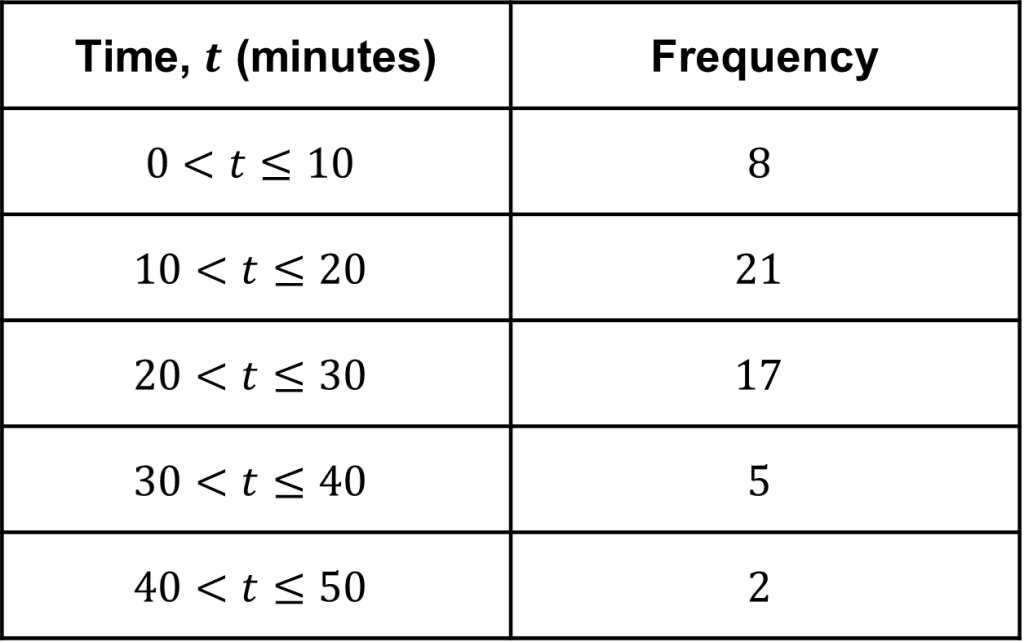The table on the left shows the time (in minutes) taken for some students to complete their maths homework.

As we can see, the class widths are equal, with all the times being categorised into $10$ minute intervals.

Looking at the grouped frequency table, we can see that it took $5$ students more than $30$ minutes but less than or equal to $40$ minutes to complete their maths homework.

Using the data, we are able to find the modal class, or in other words the time interval in which the most students completed their homework within.

In this case, the modal interval is $10

We cannot make a more accurate statement for the mode of the data, as we don’t know the specific times it took each student to complete their homework.

Level 4-5GCSEKS3AQAEdexcelOCRWJEC

## Grouped Frequency Tables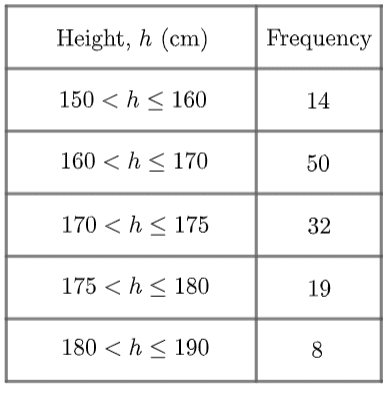This particular example is a set of data on the heights of a selection of adults. To understand what this table is saying, let’s look at the first group (also known as a class):

$150

This notation means that all people who fall within this group are

“taller than $150$ cm but no taller than $160$ cm.”

It’s important to understand that this group doesn’t include people who are exactly $150$ cm tall, but it does include people who are exactly $160$  cm tall.

This distinction matters, because we have to be clear about which group people on the edges of their class will fit into.

So, we know there are $14$ people who are taller than $150$ cm but no taller than $160$ cm. We don’t know how tall each of those $14$ people were, only that they fall in that group. This is the disadvantage of grouped frequency tables.

Level 4-5GCSEKS3AQAEdexcelOCRWJECEdexcel iGCSE

## Example: Grouped Frequency ModeThe grouped frequency table below shows data on the weights of $117$ cats.

Find the modal class.

[1 mark]

Modal class: Here we must find the class which has the highest frequency – this is the modal class. Here, this is $3.5 < w \leq 4$.

Level 4-5GCSEKS3AQAEdexcelOCRWJEC

## Example: Grouped Frequency Median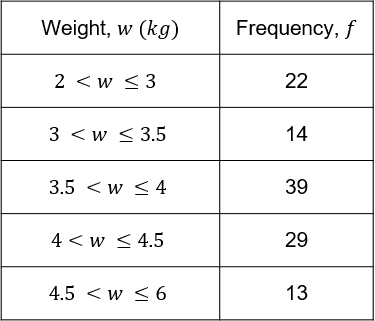The grouped frequency table below shows data on the weights of $117$ cats.

Find the class containing the median.

[2 marks]

There are $117$ cats in total, so the median is the $\dfrac{117 + 1}{2} = 59\text{th}$ cat.

Since $22+14=36<59$ and $22+14+39=75>59$, the median class must be $3.5.

Level 4-5GCSEKS3AQAEdexcelOCR

## Grouped Frequency Tables Example Questions

There are several good ways to do this question, one of which is to cross off each number as you go, and tally them in the appropriate groups.

For clarity, here is a full list of heights for each group.

The $0 group:

$7,9,15,19,19$

The $20 group:

$21,22,25,25,27,28,30$

The $30 group:

$31,32,32,33,35,37,38,39$

The $40 group:

$46,51,55,61$

So, the completed table should look like this: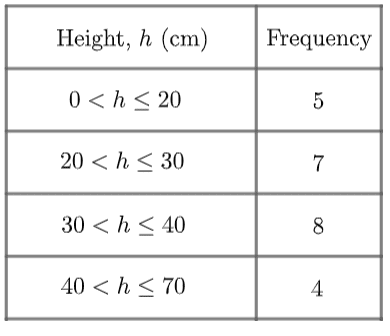Gold Standard Education

a) The bottom two groups in the table amount to the total number of people who took over $2$ minutes. Therefore, the total number of people who took over 2 minutes to solve the puzzle is:

$19+19=38\text{ people}$

b) First of all, we need to convert $90$ seconds to minutes.

$90\div60=1.5\text{ minutes}$

We now need to look for the number of people who took up to $1.5$ minutes to solve the test. The first two groups in the table represent the people who completed the puzzle in under $90$ seconds, so the total can be calculated as follows:

$8+22=30\text{ people}$

If $30$ people out of $100$ completed the puzzle in under $90$ seconds, then this can be written as the following fraction:

$\dfrac{30}{100}$

This fraction can be simplified to:

$\dfrac{3}{10}$Gold Standard Education

a)  Probably the easiest way to complete the frequency table is to use tally marks, crossing off each mark as you go through the list.

The completed frequency table should like this: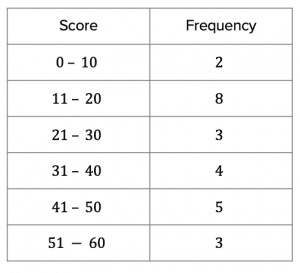b)  This question is asking for the percentage of students who scored above 40 (that is 41 marks or more).  We can see from the frequency table that 5 students scored between 41 and 50 marks and 3 students scored between 51 and 60 marks.  Therefore, 8 students in total scored above 40.

Since there are 25 students in total, of which 8 scored higher than 40, we can write this as the following fraction:

$\dfrac{8}{25}$

We need to convert this to a percentage.  To do this (if you are unsure) divide the numerator by the denominator and multiply by 100, as follows:

$8\div25\times100 = 32\%$Gold Standard Education

a)  Probably the easiest way to complete the frequency table is to use tally marks, crossing off each name as you go through the list.

The completed frequency table should like this: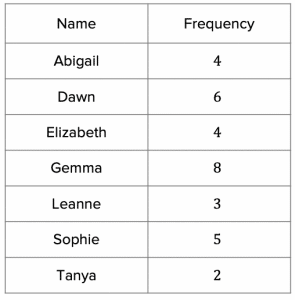b)  If we add up the totals in the frequency column, this grand total will represent the total number of students in the form group:

$4+6+4+8+3+5=32\text{ students}$

If 8 out of the 32 students voted for Gemma, this can be written as the following fraction:

$\dfrac{8}{32}$

This fraction can be simplified to:

$\dfrac{4}{16}$

This can again be simplified to:

$\dfrac{2}{8}$

This can again be simplified to:

$\dfrac{1}{4}$

Therefore, if $\frac{1}{4}$ of the students voted for Gemma, then $\frac{3}{4}$ of the students did not vote for her.Gold Standard Education

a)  Probably the easiest way to complete the frequency table is to use tally marks, crossing off each time as you go through the list.

The completed frequency table should like this: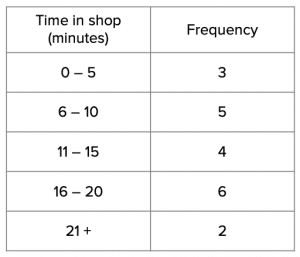b)  From our frequency table, we need to find the rows that correspond to customers who were in the shop for 10 minutes or more.

4 people spent between 11 and 15 minutes, 6 people spent between 16 and 20 minutes and 2 people spent over 20 minutes.

Therefore the number of people who spent more than 10 minutes in the bike shop is $4+6+2=12$ customers.

If their average spend was £12.50 each, then to work out the total spent by these 12 customers, we simply need to multiply this amount by 12:

$\pounds12.50\times12 = \pounds150$

c)  We are looking for customers who spent more than 10 minutes, but less than 21 minutes, so we need to add together the total from the 11 – 15 minute row and the 16 – 20 minute row.  This comes to a total of $4+6=10$ customers.

If we add up the frequency column we know that in total there were $3+5+4+6+2=20$ customers in total.  If 10 out of the 20 customers spent more than 10, but less than 21 minutes, then this cam be written as the following fraction:

$\dfrac{10}{20}$

This fraction can easily be simplified to:

$\dfrac{1}{2}$Gold Standard Education

## Grouped Frequency Tables Worksheet and Example Questions

### (NEW) Grouped Frequency Tables Exam Style Questions

Level 4-5GCSENewOfficial MME

## Grouped Frequency Tables Drill Questions

### Averages From Frequency Tables - Drill Questions

Level 4-5GCSEGold Standard Education

Level 4-5GCSE

Level 4-5GCSE

Level 4-5GCSE